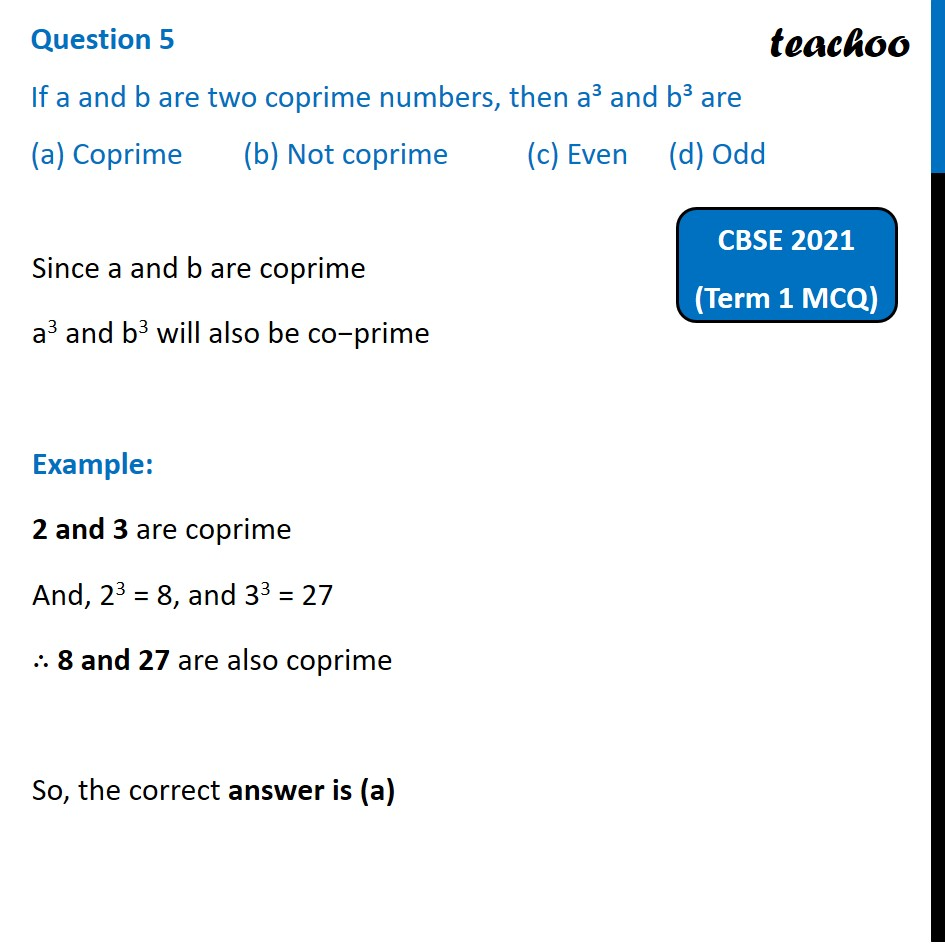Past Year MCQ (Maths Standard)

Chapter 1 Class 10 Real Numbers
Serial order wiseGet live Maths 1-on-1 Classs - Class 6 to 12

### Transcript

Question 5 If a and b are two coprime numbers, then a³ and b³ are (a) Coprime (b) Not coprime (c) Even (d) OddSince a and b are coprime a3 and b3 will also be co−prime Example: 2 and 3 are coprime And, 23 = 8, and 33 = 27 ∴ 8 and 27 are also coprime So, the correct answer is (a)